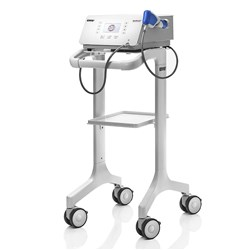# Electrotherapy

Grid List
\$21.36
Increase value Decrease value
\$21.25
Increase value Decrease value
\$47.25
Increase value Decrease value
\$579.00
Increase value Decrease value
\$849.00
Increase value Decrease value
\$1250.00
Increase value Decrease value
\$1725.00
Increase value Decrease value
\$56.00
Increase value Decrease value
\$56.00
Increase value Decrease value
Intelect Mobile Carry Bag
\$210.00
Increase value Decrease value
Intelect Therapy Cart
\$621.50
Increase value Decrease value
\$1373.90
Increase value Decrease value
\$2420.00
Increase value Decrease value
\$21.90
Increase value Decrease value
\$21.80
Increase value Decrease value
\$21.80
Increase value Decrease value
\$194.85
Increase value Decrease value
\$194.85
Increase value Decrease value
Compex Cables 1 set of 4 Snap Cables
\$162.80
Increase value Decrease value
Compex Gel
In Stock
\$24.00
Increase value Decrease value
Compex Charger (Wireless Models)
\$158.40
Increase value Decrease value
\$45.05
Increase value Decrease value
\$19.55
Increase value Decrease value
\$20.90
Increase value Decrease value
\$19.65
Increase value Decrease value
\$20.90
Increase value Decrease value
\$25.75
Increase value Decrease value
\$19.95
Increase value Decrease value
\$97.90
Increase value Decrease value
Compex Battery
In Stock
\$150.43
Increase value Decrease value
\$20.90
Increase value Decrease value
\$32.50
Increase value Decrease value
\$63.36
Increase value Decrease value
Valutrodes Electrodes 3.2cm Round (4)
\$14.70
Increase value Decrease value
Valutrodes Electrodes 5cm Round (4)
\$12.20
Increase value Decrease value
\$12.20
Increase value Decrease value
\$22.50
Increase value Decrease value
In Stock
\$22.22
Increase value Decrease value
\$14.50
Increase value Decrease value
EMS Swiss Dolorclast Smart20 Evo Shockwave
\$28160.06
Increase value Decrease value
EMS Swiss Dolorclast Master Touch Evo Shockwave
\$36249.10
Increase value Decrease valueEMS Swiss Dolorclast Portable Radial Shockwave
\$27445.00
Increase value Decrease value
\$527.00
Increase value Decrease value
\$259.00
Increase value Decrease value
\$250.00
Increase value Decrease value
Neurotrac TENS
In Stock
\$240.00
Increase value Decrease value
\$1175.00
Increase value Decrease value
Nu-Tek Combination Unit
\$3168.00
Increase value Decrease value
Grid List
##### Products to compare:
Comparing Products